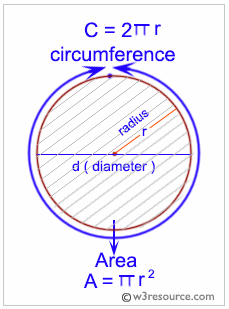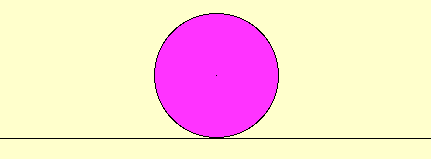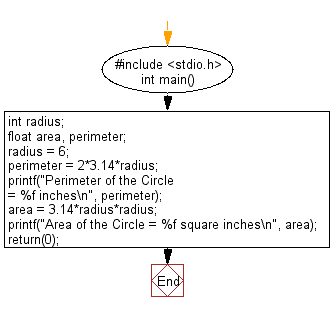﻿ C Program: Compute the perimeter and area of a circle - w3resource

# C Exercises: Compute the perimeter and area of a circle

## C Basic Declarations and Expressions: Exercise-6 with Solution

Write a C program to compute the perimeter and area of a circle with a given radius.

C programming: Area and circumference of a circle

In geometry, the area enclosed by a circle of radius r is πr2. Here the Greek letter π represents a constant, approximately equal to 3.14159, which is equal to the ratio of the circumference of any circle to its diameter.

The circumference of a circle is the linear distance around its edge.

Pictorial Presentation:Why is the area of a circle of a circle pi times the square of the radius?C Code:

``````#include <stdio.h>

int main() {
int radius;      /* Variable to store the radius of the circle */
float area, perimeter;    /* Variables to store the area and perimeter of the circle */

/* Calculating the perimeter of the circle */
perimeter = 2 * 3.14 * radius;
printf("Perimeter of the Circle = %f inches\n", perimeter);

/* Calculating the area of the circle */
printf("Area of the Circle = %f square inches\n", area);

return(0);
}
``````

Explanation:

In the exercise above -

• The program includes the standard input/output library <stdio.h>.
• It declares several variables:
• float area and float perimeter: Will store the calculated area and perimeter of the circle.
• In the "main()" function:
• It assigns the value 6 to the 'radius' variable, representing the circle radius.
• The program then calculates the circle's perimeter and area:
• Perimeter: It uses the formula 2*3.14* radius to calculate the circle perimeter and stores the result in the 'perimeter' variable. Here, 3.14 is an approximation of the mathematical constant π (pi).
• Area: It calculates the area using the formula 3.14*radius*radius (π * r^2) and stores the result in the 'area' variable.
• Finally, the program uses the "printf()" function to display the calculated values:
• It prints the calculated perimeter with a message: "Perimeter of the Circle = [perimeter] inches."
• It prints the calculated area with a message: "Area of the Circle = [area] square inches."
• The program returns 0 to indicate successful execution.

Sample Output:

```Perimeter of the Circle = 37.680000 inches
Area of the Circle = 113.040001 square inches
```

Flowchart:C Programming Code Editor:

What is the difficulty level of this exercise?

Test your Programming skills with w3resource's quiz.

﻿